# Place Value, Rounding, Addition, and Subtraction

## Objective

Locate multi-digit numbers on a number line and explain their placement.

## Common Core Standards

### Core Standards

?

• 4.NBT.A.2 — Read and write multi-digit whole numbers using base-ten numerals, number names, and expanded form. Compare two multi-digit numbers based on meanings of the digits in each place, using >, =, and < symbols to record the results of comparisons.

• 4.NBT.A.3 — Use place value understanding to round multi-digit whole numbers to any place.

?

• 2.MD.B.6

## Criteria for Success

?

1. Understand that a number line is a straight line with equally spaced intervals used to plot numbers.
2. Understand that number lines help to visually show the relationship between numbers.
3. Identify the location of a point on various number lines, including:
• Those with and without intervals (i.e., “open” number lines)
• Those with intervals marked by not labeled
• Those not starting at 0
• Those with intervals greater than 1
1. Plot a point on various number lines that may or may not have labeled tick marks and/or intervals.

#### Fishtank Plus

• Problem Set
• Student Handout Editor
• Vocabulary Package

?

### Problem 1

1. Look at the line below: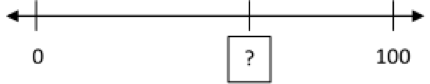Based on where 0 and 100 are, what number do you think the question mark is on? Explain your choice.

1. Look at the line below: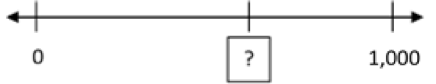Based on where 0 and 1,000 are, what number do you think the question mark is on?

#### References

John A. Van de Walle Teaching Student-Centered Mathematics: Developmentally Appropriate Instruction for Grades 3-5 (Volume II)Activity 10.2

Van de Walle, John A. Teaching Student-Centered Mathematics: Developmentally Appropriate Instruction for Grades 3-5 (Volume II). Pearson, 2nd edition, 2013.

Modified by The Match Foundation, Inc.

### Problem 2

1. What is the location of the following point?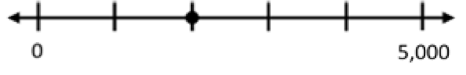1. What is the location of the following point?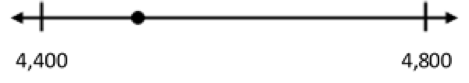### Problem 3

1. Plot 3,756 on a number line from 3,750 to 2,760.
2. Plot 3,756 on a number line from 3,700 to 3,800.
3. Plot 3,756 on a number line from 3,000 to 4,000.

## Problem Set & Homework

#### Discussion of Problem Set

• What’s different about the values in #2(a) and (b)? How is that difference represented on the number line?
• Look #3. How did you decide what to make your number lines look like to place 156,780 on them? How do your number lines look similar and different (to each other and to other students’ number lines)?
• Look at #6. How did you determine where each value would go?
• What values fit both criteria in #9? How did you figure that out?

?

1. Label all the other tick marks between 8,260 and 8,270. Then plot 8,263.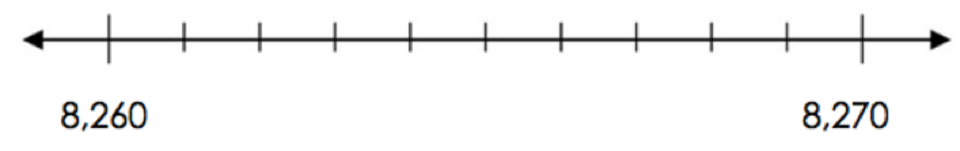1. Plot 8,200 and 8,300 on the two outermost spots on the number line below. Label the rest of the tick marks. Then plot 8,263.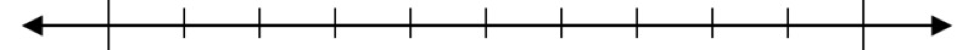1. Plot 8,000 and 9,000 on the two outermost spots on the number line below. Label the rest of the tick marks. Then plot 8,263.### Mastery Response

?